Home | | Maths 10th Std | Exercise: Relation and Function

# Exercise: Relation and Function

Maths Book back answers and solution for Exercise questions - Mathematics : Relation and Function: Exercise Questions with Answer

UNIT EXERCISE

1. If the ordered pairs (x2  − 3xy2  + 4y) and (-2, 5) are equal, then find and y.2. The cartesian product A×has 9 elements among which (–1, 0) and (0,1) are found. Find the set and the remaining elements of A×A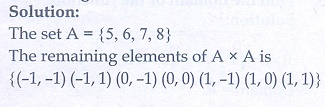3. Given that (x) =(i) f (0)

(ii) (3)

(iii) (+ 1) in terms of a.(Given that ≥ 0 )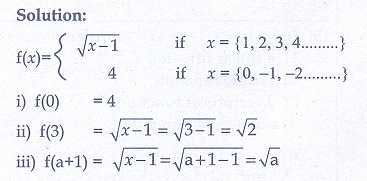4. Let = {9,10,11,12,13,14,15,16,17} and let f→ N be defined by (n) = the highest prime factor of  . Write as a set of ordered pairs and find the range of f.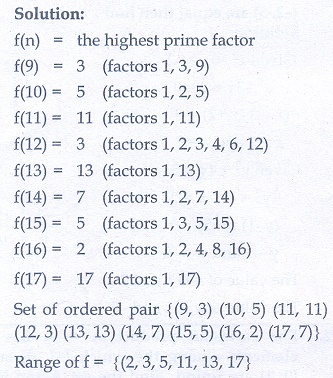5. Find the domain of the function (x) =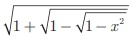6. If (x) = x2 , g(x) = 3and h(x) = − 2 , Prove that (o go o (o h)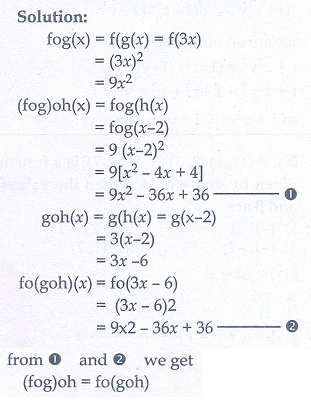7. Let = {1, 2} and = {1, 2, 3, 4} , C  = {5, 6} and = {5, 6, 7, 8} . Verify whether A×C is a subset of B×D?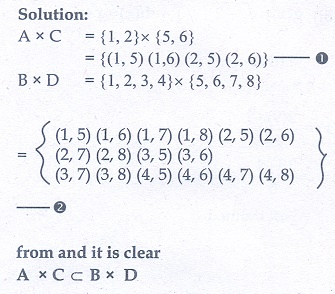8. If  (x= [x-1]/[x+1], x ¹ 1 show that ((x)) = −1/x provided x ≠ 0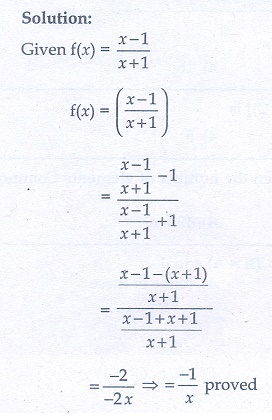9. The functions and are defined by (x) = 6+ 8; g(x) = [x-2]/3

(i). Calculate the value of gg(1/2)

(ii) Write an expression for gf (x) in its simplest form.10. Write the domain of the following real functions(iv) h(x) = + 6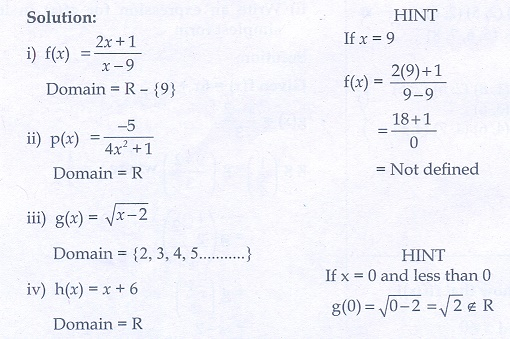1. 1,2 and -5 ,1

2. {-1, 0, 1} , {(- 1, -1),(- 1, 1),(0, -1),(0, 0),(1, -1),(1, 0),(1, 1)}

3.(i) 4 (ii) √2 (iii) √a

4. {(9, 3),(10, 5),(11, 11),(12, 3),(13, 13),(14, 7),(15, 5),(16, 2),(17, 17)} , {2,3,5,11,13,17}

5. {–1,0,1}

9.(i) -5/6 (ii) 2(x + 1)

10.(i) R - {9} (ii) R (iii) [2, ∞) (iv) R

Tags : Problem Questions with Answer, Solution | Mathematics , 10th Mathematics : UNIT 1 : Relation and Function
Study Material, Lecturing Notes, Assignment, Reference, Wiki description explanation, brief detail
10th Mathematics : UNIT 1 : Relation and Function : Exercise: Relation and Function | Problem Questions with Answer, Solution | Mathematics